KMP 算法的理解10
2018/10

# KMP 算法的理解

## BP 算法

• 主串 S 和 子串 T ，且 S.length > T.length
• 串的物理位置从0开始

• 从初始位置开始与子串完全匹配 -> 匹配成功
• 在 主串在 i 的位置上匹配失败（此时 子串位置为 j） -> 主串从第二个位置与子串的第一个字符重新开始匹配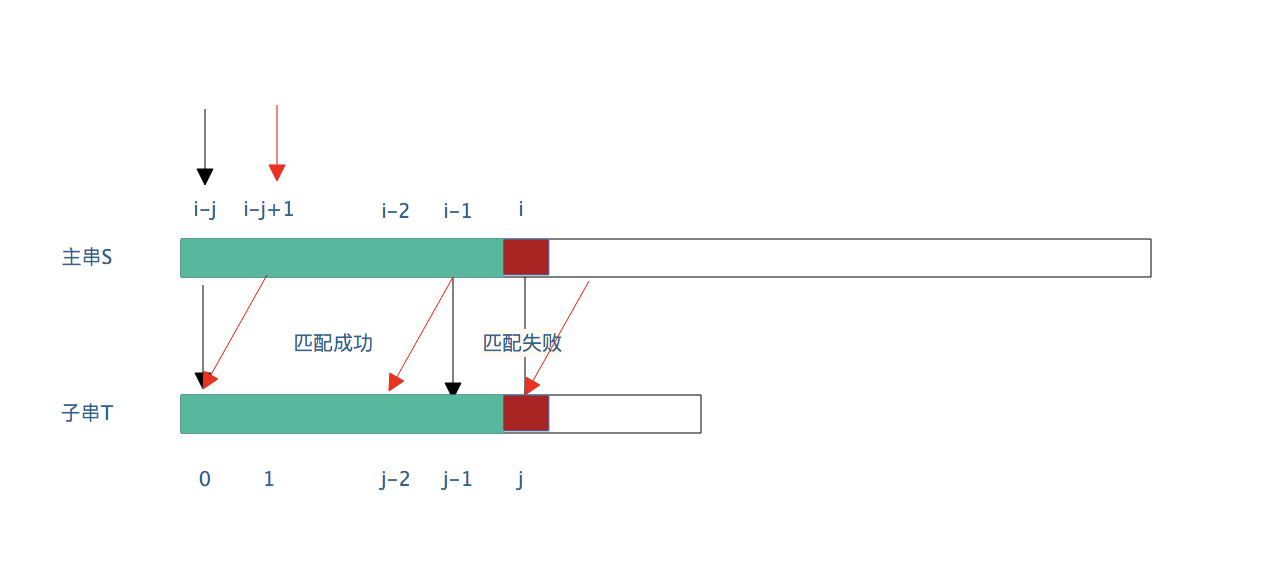``````int index(String s, String t){

for (int i = 0,j=0;i<s.length && j < j.length;){
if (s[i] == t[j]){//目前匹配成功
j++;
i++;
}else{//该位置匹配失败，i需要返回到一开始的位置并加1重新检测匹配
i = i - j + 1;
j = 0;
}
}

//结束循环后，判断是否匹配成功
if (j >= t.length){//表示j已经访问了整个t子串了，即匹配成功
return i - j - 1; // 返回一开始成功匹配的位置
}else{
return -1;
}
}``````

• 这里的“对称性（相似性）”并不是一般的对称（一般的对称如某个图像关于X轴对称，这样沿着X轴折叠，两边可以重叠）。如下图所示：• 举一些例子： 比如 子串 `abab`，相似的区域是 `ab`

1. 大部分的串或多或少都有一些相似性，如果没有任何“相似性”，BP算法仍然有优化空间（根据子串的不相似性减少不必要的检查，这个KMP部分会解释）
2. 子串的两个相似区域不能完全重叠，必须偏移一定的距离。比如子串 `aaaa`，相似区域是`aaa`（相似区域不是`aaaa`，因为不能完全重叠）。

KMP算法的核心就是：

1. 寻找模式子串每个字符前面的"小子串"的相似区域的长度。
2. 利用这个相似区域减少不必要的检查匹配。

## KMP 算法

KMP算法能够优化寻找过程的依赖的性质有两点：

1. 子串匹配失败处前面的“小子串”与上面的主串对应部分是完全相等的
2. “小子串”具有“相似性”，即有相似区域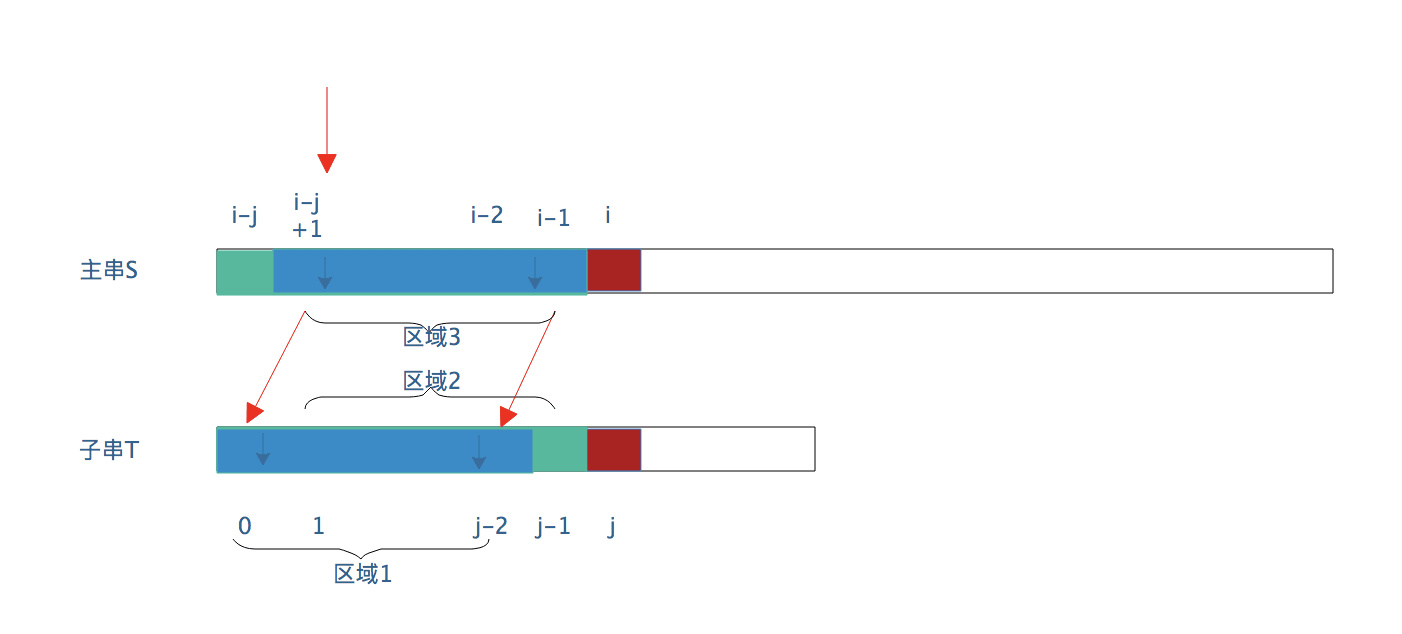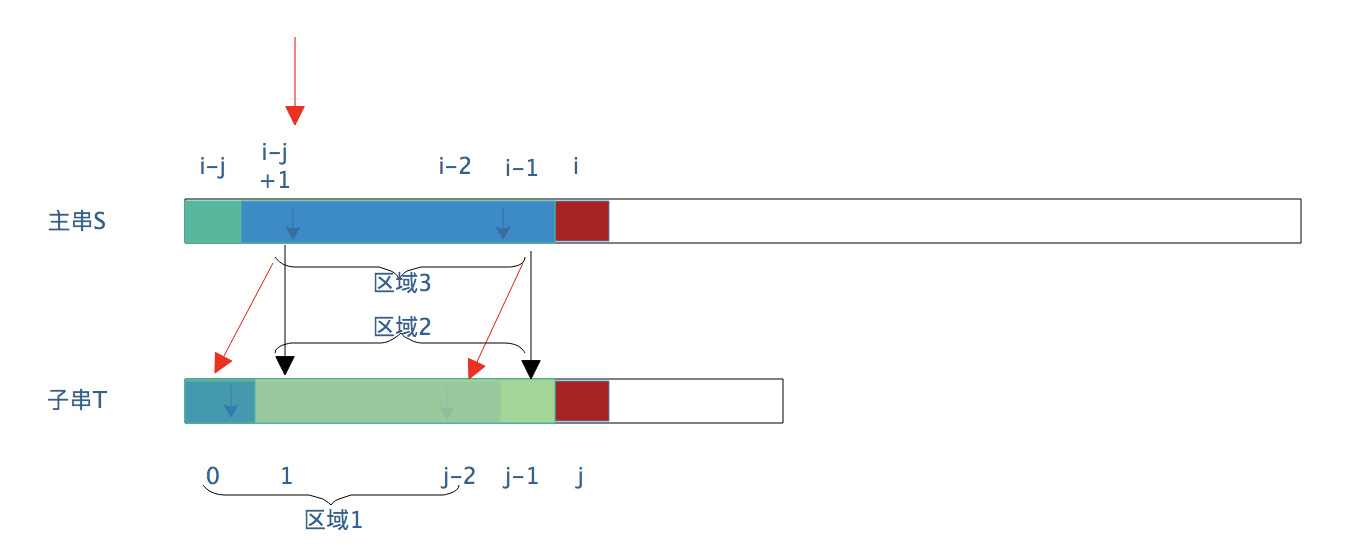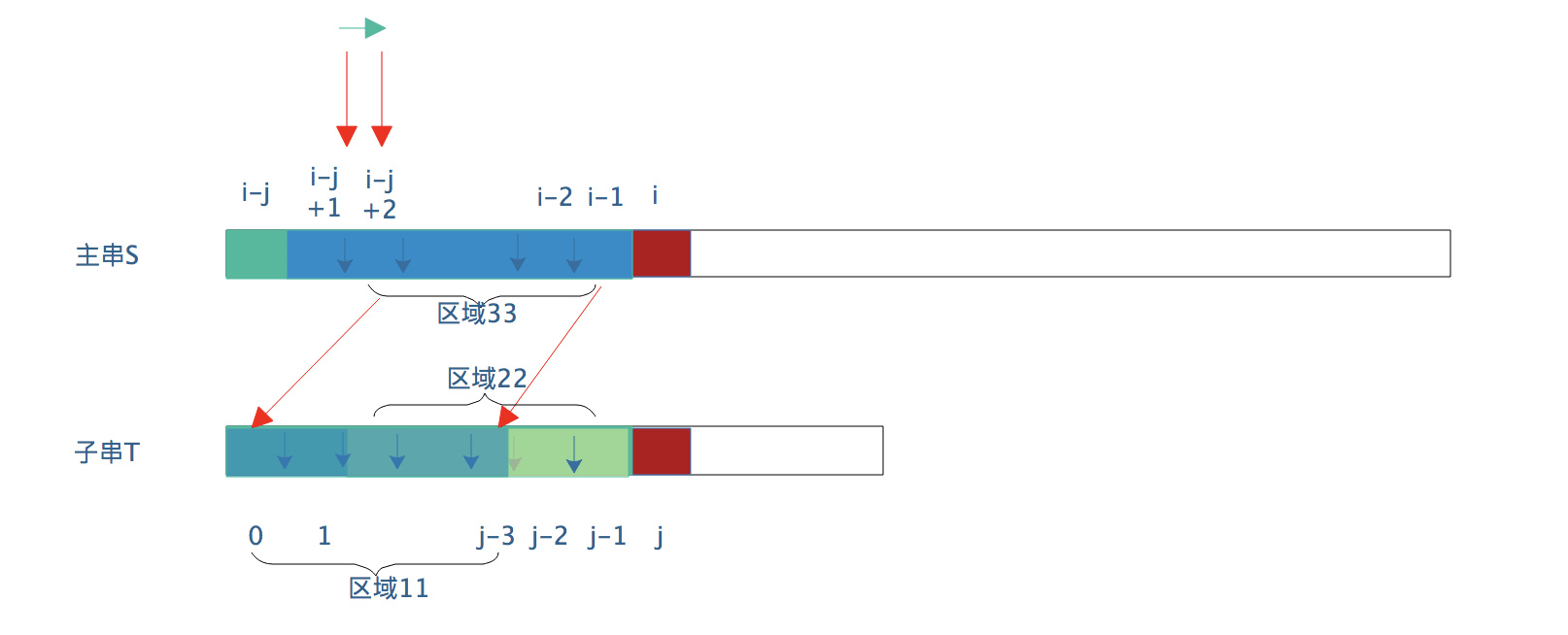……

1. 主串的 i 指针不需要返回
2. s[i] 与 t[k] 直接比较，这里的k是小子串相似区域的长度。这个k的意义是，当子串j位置匹配失败，下一步子串j指针需要移动到k的位置上（j = k）然后s[i]与t[k]开始检测匹配。

1. 获取到模式子串的next数组
2. 当匹配失败时，主串i指针不需要返回，模式子串j 移动到next[j]的位置，继续进行匹配检测，直到主串检测完毕（i>= s.length）

1. （固定前两位的值）next = -1;next  = 0;
2. 然后从j=2开始，求出该位置前面的小子串的相似区域的长度即可。

``````if (j == -1){// 前面已经做了j = next[j]，具体看下面完整代码
i++;// 主串 i 指针前移一位
j++;// j 变成0，即与t进行匹配检测
}``````

``````int KMPindex(String s,String t){
//1. 求出子串的next数组
int [] next = new int[MaxSize];
next = -1;next = 0;//固定的前两位的值
int k = -1;
for(int j= 0;j<t.length;){//对每一位找到小子串的相似区域的长度
// 手算next数组很简单的，但是用代码则不容易。李春葆《数据结构》上的代码非常精妙，仅用4行
// 如果按照课本上的代码过程发现确实能得到正确结果，但是自己根本想不到这么写啊
// 考研基本不考这部分代码，我先不深入研究了，会手算即可

if (k == -1 || t[j] == t[k]){
j++;
k++;
next[j] = k;
}else{
k = next[k];
}
}

//2. 开始匹配,可以看到匹配过程代码和BP算法基本一样，只是在匹配出错的地方处理不一样
for (int i = 0,j=0;i<s.length && j < j.length;){
if (j == -1 || s[i] == t[j]){//目前匹配成功 或者 匹配失败且j = (next[j] == -1)
j++;
i++;
}else{//该位置匹配失败，i不动，j移动到next[j]的位置继续与s[i]比较
j = next[j];
}
}

//结束循环后，判断是否匹配成功
if (j >= t.length){//表示j已经访问了整个t子串了，即匹配成功
return i - j - 1; // 返回一开始成功匹配的位置
}else{
return -1;
}
}``````

``````//2. 开始匹配,可以看到匹配过程代码和BP算法基本一样，只是在匹配出错的地方处理不一样
for (int i = 0,j=0;i<s.length && j < j.length;){
if (s[i] == t[j]){//目前匹配成功
j++;
i++;
}else{//该位置匹配失败，i不动
if(next[j] != -1){//j移动到next[j]的位置继续与s[i]比较
j = next[j];
}else{//如果next[j] == -1，表示该匹配失败处是子串的0位置，所以i需要前移一位，j仍然保持不变为0
j = 0;//这句话可以去掉，因为此时j一定为0
i ++;
}
}
}

//结束循环后，判断是否匹配成功
if (j >= t.length){//表示j已经访问了整个t子串了，即匹配成功
return i - j - 1; // 返回一开始成功匹配的位置
}else{
return -1;
}``````

KMP算法的问题在于忽视了一种特殊的模式子串，如`aaaaa`，这种子串还能够利用本身的特殊值相等性质进一步简化检测匹配的过程。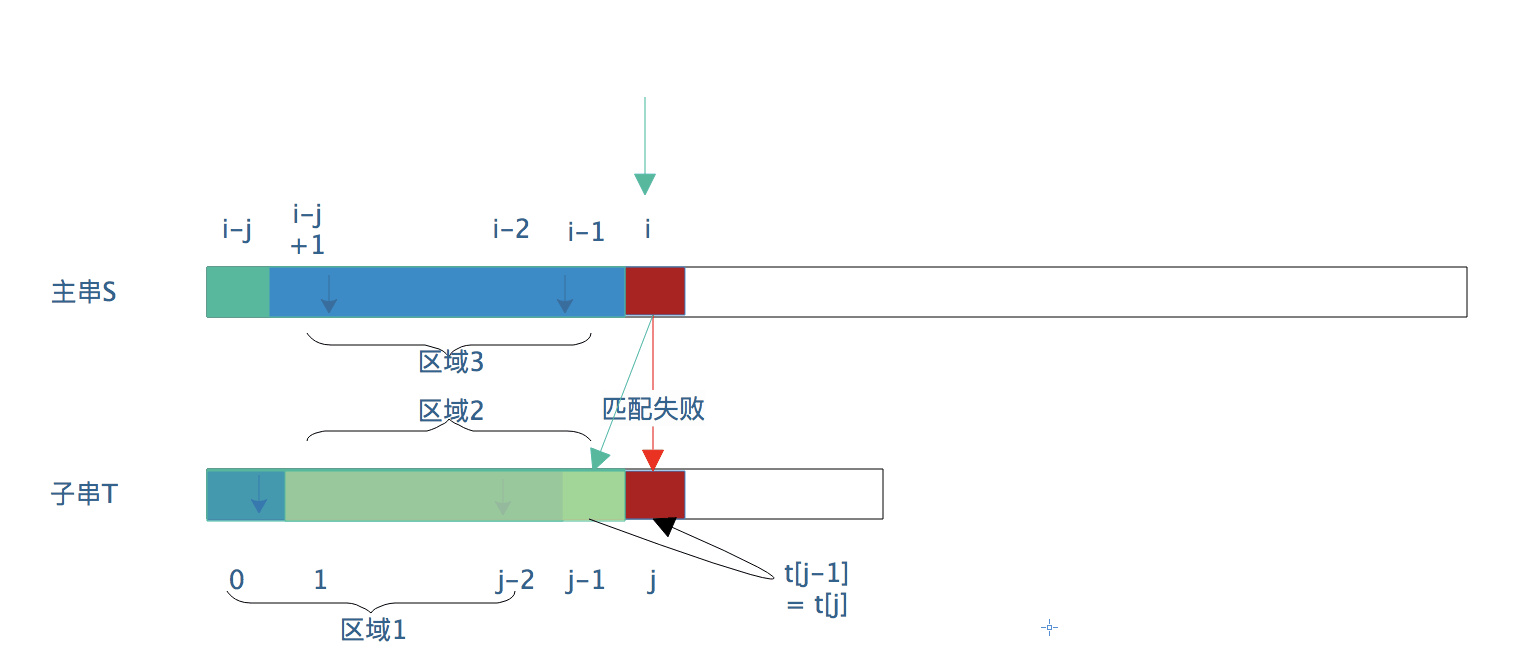## KMP 改进算法

• 如果相等，next[j] =next[next[j]];
• 如果不相等话，next[j] 值还是以前的计算方法（小子串相似区域的长度）

• 如果相等，next[j] =nextval[next[j]];

• 如果不相等话，nextval[j] = next[j]

KMP改进算法的代码：

``````int KMPindex(String s,String t){
//1. 求出子串的nextval数组
int [] nextval = new int[MaxSize];
nextval = -1;//固定的前一位的值
int k = -1;
for(int j= 0;j<t.length;){//对每一位找到小子串的相似区域的长度
//同KMP,只要求手算结果即可，代码没有深入研究
}

//2. 开始匹配,可以看到匹配过程代码和BP算法基本一样，只是在匹配出错的地方处理不一样
for (int i = 0,j=0;i<s.length && j < j.length;){
if (s[i] == t[j]){//目前匹配成功
j++;
i++;
}else{//该位置匹配失败，i不动
if(next[j] != -1){//j移动到nextval[j]的位置继续与s[i]比较
j = nextval[j];
}else{//如果nextval[j] == -1，表示j最终会移动到0位置上，且 t[j] == t，所以
j = 0;//这句话一定不能去掉！！因为不仅只有0位置nextval = -1还有别的位置也可能等于-1
i ++;
}
}
}

//结束循环后，判断是否匹配成功
if (j >= t.length){//表示j已经访问了整个t子串了，即匹配成功
return i - j - 1; // 返回一开始成功匹配的位置
}else{
return -1;
}
}``````

## 最后

1. 代码是在编辑器手敲的，没有经过代码运行，很大可能是运行有错误，只是用来展示算法思路
2. 可以看到KMP（以及改进后）算法本质上都是在处理子串j位置匹配失败，子串的j需要如何移动。所以next(nextval)数组的意义就是如果该位置匹配失败了，当前位置应该移动到什么位置上
3. KMP算法和BP算法在匹配成功的过程中是完全一致的
4. 理解可能有错误之处，请不吝指正

#### 12 条评论

1.一名路过的码农

请问博主用的是啥编辑器呀，可以随时截图本地上传吗？

2.谁知道呢

博主学过编译原理就应该很容易明白kmp算法的原理了，其实用自动机的角度解释kmp会更清楚。

3.mofanrs

作者你好你的主题有同步到熊掌号这个功能OωO吗

4.IamMicroCoder大佬儿

#### 发表评论